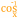# Arc Cosine

 Block Group: Trigonometric Functions Icon:The Arc Cosine block returns the arccosine, or inverse cosine, of a number, in degrees. The arccosine of n is the angle for which n is the cosine.

For information on using dataflow blocks, see Dataflow.

## Input/Output Property

The following property of the Arc Cosine block can take input and give output.

• input (number)

input defines the cosine to get the angle for. Must be between −1 and 1.

## Output Property

The following property of the Arc Cosine block can give output but cannot take input.

• output (number)

output returns the arccosine of the input, in degrees.

## Example

The following image shows an example of the Arc Cosine block. In this example, the cosine is –1, and the arccosine is 180 degrees.